## Korg Nutube 6P1 Hybrid Amplifier circuit test and verification

Below a close picture of the Korg NuTube 6P1 powered on.Korg Nutube 6P1
A picture of the whole board powered on.
Blue LED is +24V power on, greeen LED is +5V power on.Top sliding switch is +24V on/off, just below is the +5V on/off switch. To the right is the Gain Boost on/off for the power amp 8ohm speaker section, just below, the blue potentiometer, is the output gain control, below is the Gain Boost switch for the headphones/line-out section, below it is the power amp volume, below at the bottom is the headphones/line-out volume.
On the left at the bottom are the three controls for the 3-band equalizer, based on a Fender tone stack: bass, mid and trebles. Above the bass control is the input gain.
The 24-pins TSSOP device on the right is the 25W class-D power amplifier.
NuTube 6P1 is plugged into a 2mm pitch socket resting over 2 rubber bumpers to avoid vibrations that could pick microphonic noise.Korg Nutube 6P1 Hybrid 25W Amplifier

### Wave signal verification with oscilloscope

Summary of results:
• First JFET amplifier gain with gain control
• G1=0.0 =>-2.7 dB
• G1=0.5 =>  0.1 dB
• G1=1.0 =>  1.6 dB
• First NuTube 6P1 triode stage gain is 14dB
• Second JFET buffer gain is 0dB
• 3-band equalizer gain at 1kHz with Bass, Mid and Top = 0.5 is -18dB
• Second NuTube 6P1 triode stage gain is 15dB
• Third JFET amplifier gain with gain control and Boost Off:
• G2=0.0 =>-20 dB
• G2=0.5 => -5 dB
• G2=1.0 => -1 dB
• Third JFET amplifier gain with gain control and Boost ON:
• G2=0.0 =>-2.5 dB
• G2=0.5 => 12 dB
• G2=1.0 => 15 dB
Maximum output level is 12750mVpp which corresponds to a maximum total gain of 26.5dBInput signal: Sine wave 600mVpp 1kHz
A 1kHz sinewave 600mVpp is used at the input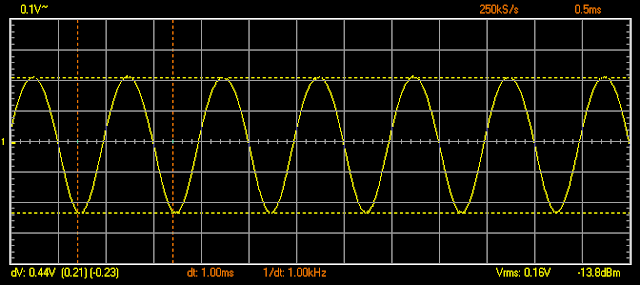TP2 signal after first JFET buffer 440 mVpp. Gain 1 = 0.0 (-2.7 dB)TP2 signal after first JFET buffer 610 mVpp. Gain 1 = 0.5. (0.1 dB)TP2 signal after first JFET buffer 720 mVpp. Gain 1 = 1.0 (1.6 dB)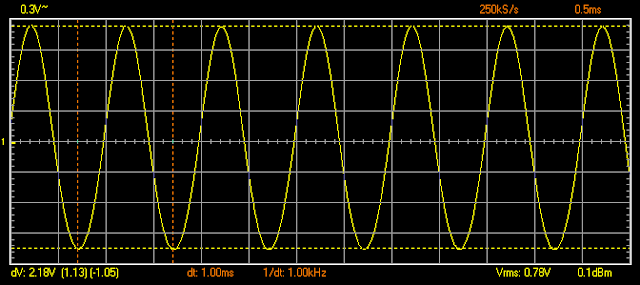TP4 signal after first triode anode 2180mVpp. Gain 1 = 0.0 (13.9 dB)TP4 signal after first triode anode 2910mVpp. Gain 1 = 0.5 (13.6 dB)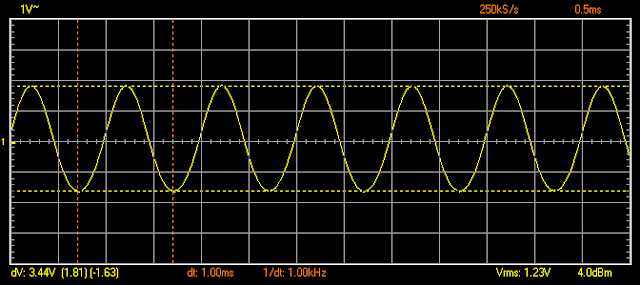TP4 signal after first triode anode 3440mVpp. Gain 1 = 1.0 (13.6 dB)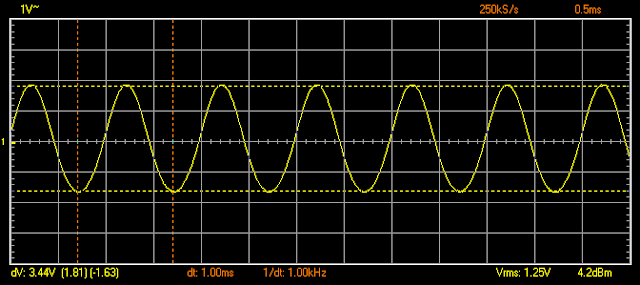C16 signal after second JFET buffer 3440mVpp. Gain 1 = 1.0 (0 dB)C20 signal after 3-band equalizer 450mVpp. Gain = 1.0, Treble=Middle=Bass = 0.5  (-17.7 dB)TP21 signal after third JFET buffer 380mVpp (-1.5 dB)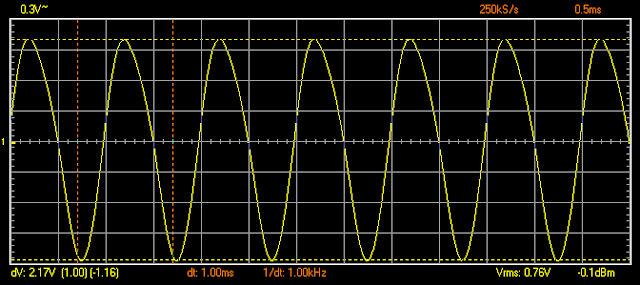TP5 signal after second triode anode 2170mVpp (15.1 dB)C27 signal after fourth JFET amplifier 220mV, Gain 2 = 0 (-20 dB)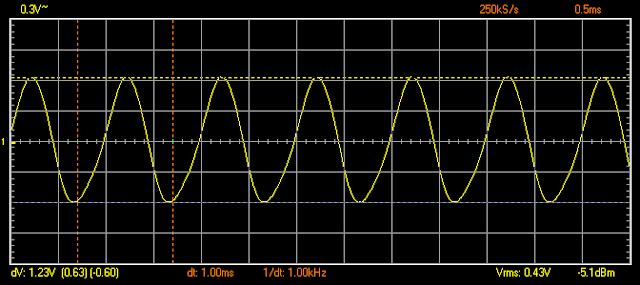C27 signal after fourth JFET amplifier 1230mV, Gain 2 = 0.5 (-5 dB)C27 signal after fourth JFET amplifier 1950mV, Gain 2 = 1.0 (-1 dB)C27 signal after fourth JFET amplifier 1620mV, Gain 2 = 0.0. BOOST ON (-2.5 dB)C27 signal after fourth JFET amplifier 8810mV, Gain 2 = 0.5. BOOST ON (12.2 dB)C27 signal after fourth JFET amplifier 12750mV, Gain 2 = 1.0. BOOST ON (15.4 dB)